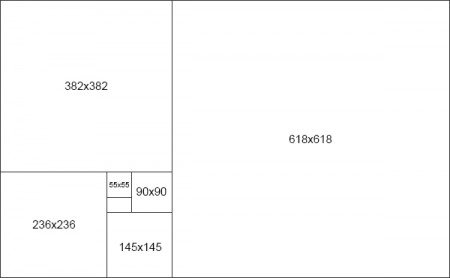## Using the Golden Ratio in Design

September 29, 2019 / Inspiration

Using design to make your marketing material stand out is an excellent way to get noticed. However, the design needs to be good if you want to be effective. Harmony is required if you’re going to have a good design. Meaning all of the design elements are arranged effectively. One way to achieve balance is by using the golden ratio in design. The golden ratio can be found in nature, our solar system, our body, the DNA chain, music, art, design, and architecture. In fact, the Great Pyramids of Giza and the Parthenon were both built around the golden ratio, and look how well they have survived throughout the years!

The golden ratio, also known as the golden mean and the divine proportion, is a mathematical ratio that is derived from the Fibonacci sequence. The Fibonacci sequence is a naturally occurring sequence of numbers, and it directly relates to the golden ratio. This mathematical ratio, the golden ratio, is based on the number phi, which is equal to 1.618. While the Fibonacci sequence features the number sequence 1, 1, 2, 3, 5, 8, 13, 21, 34, 55, and so forth. In this sequence, the numbers come together by adding the two preceding numbers to achieve the next number in the sequence. However, when you take a number in the sequence and divide it by its previous number, you get a result that is approximate to Phi. While the difference between the two numbers isn’t always equal to the golden ratio, it’s pretty close. For example:

### Using the Golden Ratio in Design

The golden ratio creates a sense of visual harmony and balance, making it an excellent tool to add to your designer’s toolkit. You can build your design by using the golden rectangle. To create a golden rectangle, start with the width of the rectangle and divide it by 1.618. If you had a rectangle that is 1000 pixels wide, you would divide it by 1.618 to get a height of 618. Then, you add a 618 x 618 square on the right side of the rectangle, leaving behind a 382 x 618 rectangle on the left. Taking that new rectangle, you then create another square within it, which you then divide up again. This cycle repeats, but each time you divide up your golden rectangle, it becomes apparent that the largest dividing line spirals into itself. You can use the golden rectangle as a general guideline for layout. Using this “tool” can help achieve harmony and flow within a design by building your design around the sequence. In theory, you want to focus your design elements around the center of the spiral, making the main focal point of the image at the center of the golden spiral.In addition, you can use the golden ratio when you are pairing up images that are different sizes. For example, if you had an image that is 2 inches wide and wanted to keep the size difference proportional, you would divide the 2 by 1.618. 2 by 1.618 comes out to roughly 1.2 inches. For typography, you can use the golden ratio to find out your complementary font size. To determine a font size bigger than what you’re already using, take your font size, and multiply by 1.618. For example, if you are using a 10 point font, take the 10 and multiply by 1.618, which in this case is 16.18 and that gets rounded down to 16. If you need to find the complementary text font size to go with your heading, take the font size and divide it by 1.618.